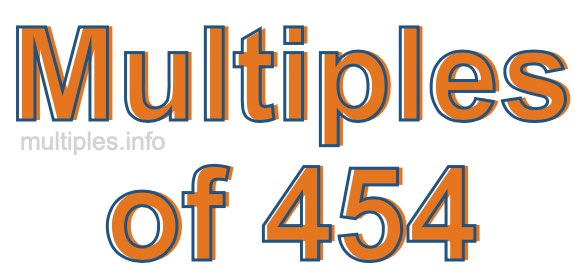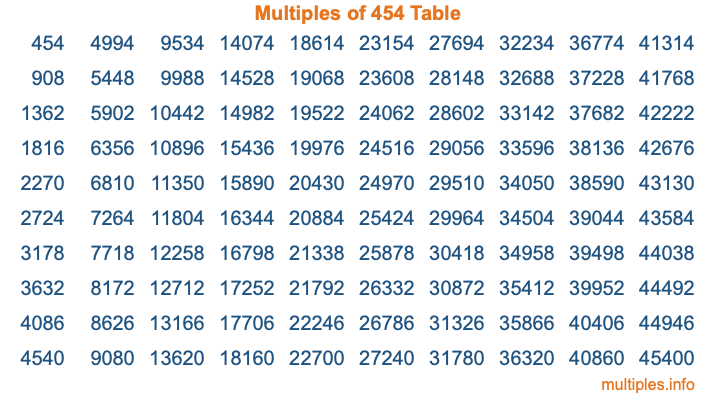Multiples of 454Welcome to the Multiples of 454 page. Here we will first teach you everything you will ever need to know about the multiples of 454, and then give you a study guide summary of everything we taught you to make sure you remember it all. Use this page to look up facts and learn information about the multiples of 454. This page will make you a multiples of four hundred fifty-four expert!

Definition of Multiples of 454
Multiples of 454 are all the numbers that when divided by 454 equal an integer. Each of the multiples of 454 are called a multiple. A multiple of 454 is created by multiplying 454 by an integer.

Therefore, to create a list of multiples of 454, you start with 1 multiplied by 454, then 2 multiplied by 454, then 3 multiplied by 454, and so on for as long as you want. Thus, the list of the first five multiples of 454 is 454, 908, 1362, 1816, and 2270. To see a larger list of multiples of 454, see the printable image of Multiples of 454 further down on this page. We also have a category where you can choose any nth multiple of 454.

Multiples of 454 Checker
The Multiples of 454 Checker below checks to see if any number of your choice is a multiple of 454. In other words, it checks to see if there is any number (integer) that when multiplied by 454 will equal your number. To do that, we divide your number by 454. If the the quotient is an integer, then your number is a multiple of 454.

Is  a multiple of 454?

Least Common Multiple of 454 and ...
A Least Common Multiple (LCM) is the lowest multiple that two or more numbers have in common. This is also called the smallest common multiple or lowest common multiple and is useful to know when you are adding our subtracting fractions. Enter one or more numbers below (454 is already entered) to find the LCM.

Check out our LCM Calculator if you need more details about the Least Common Multiple or if you need the LCM for different numbers for adding and subtraction fractions.

nth Multiple of 454
As we stated above, 454 is the first multiple of 454, 908 is the second multiple of 454, 1362 is the third multiple of 454, and so on. Enter a number below to find the nth multiple of 454.

th multiple of 454

Multiples of 454 vs Factors of 454
454 is a multiple of 454 and a factor of 454, but that is where the similarities end. All postive multiples of 454 are 454 or greater than 454. All positive factors of 454 are 454 or less than 454.

Below is the beginning list of multiples of 454 and the factors of 454 so you can compare:

Multiples of 454: 454, 908, 1362, 1816, 2270, etc.

Factors of 454: 1, 2, 227, 454

As you can see, the multiples of 454 are all the numbers that you can divide by 454 to get a whole number. The factors of 454, on the other hand, are all the whole numbers that you can multiply by another whole number to get 454.

It's also interesting to note that if a number (x) is a factor of 454, then 454 will also be a multiple of that number (x).

Multiples of 454 vs Divisors of 454
The divisors of 454 are all the integers that 454 can be divided by evenly. Below is a list of the divisors of 454.

Divisors of 454: 1, 2, 227, 454

The interesting thing to note here is that if you take any multiple of 454 and divide it by a divisor of 454, you will see that the quotient is an integer.

Multiples of 454 Table
Below is an image of the first 100 multiples of 454 in a table. The table is in chronological order, column by column. The first column has the first ten multiples of 454, the second column has the next ten multiples of 454, and so on.The Multiples of 454 Table is also referred to as the 454 Times Table or Times Table of 454. You are welcome to print out our table for your studies.

Negative Multiples of 454
Although not often discussed or needed in math, it is worth mentioning that you can make a list of negative multiples of 454 by multiplying 454 by -1, then by -2, then by -3, and so on, to get the following list of negative multiples of 454:

-454, -908, -1362, -1816, -2270, etc.

Multiples of 454 Summary
Below is a summary of important Multiples of 454 facts that we have discussed on this page. To retain the knowledge on this page, we recommend that you read through the summary and explain to yourself or a study partner why they hold true.

There are an infinite number of multiples of 454.

A multiple of 454 divided by 454 will equal a whole number.

454 divided by a factor of 454 equals a divisor of 454.

The nth multiple of 454 is n times 454.

The largest factor of 454 is equal to the first positive multiple of 454.

454 is a multiple of every factor of 454.

454 is a multiple of 454.

A multiple of 454 divided by a divisor of 454 equals an integer.

454 divided by a divisor of 454 equals a factor of 454.

Any integer times 454 will equal a multiple of 454.

Multiples of a Number
Here you can get the multiples of another number, all with the same attention to detail as we did for multiples of 454 on this page.

Multiples of
Multiples of 455
Did you find our page about multiples of four hundred fifty-four educational? Do you want more knowledge? Check out the multiples of the next number on our list!